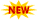MICROPROCESSOR AND MICROCONTROLLER LAB MANUAL JNTU UNIVERSITY - Computer Programming

# Computer Programming

C C++ Java Python Perl Programs Examples with Output -useful for Schools & College Students

## Thursday, October 13, 2011Sl. No            Name of Experiment

01.                   Addition of two 8 bit numbers
02.                   Addition of two 16 bit numbers
03.                   Subtraction of two 16 bit numbers
04.                   Multiplication of two 16 bit numbers
05.                   Division of 16 bit number with 8 bit number
06.                   Division of 32 bit number with 16 bit number
07.                   Addition of two BCD numbers
08.                   Sum of squares of first ‘n’ numbers
09.                   Sum of cubes of first ‘n’ numbers
10.                   The length of the given string
11.                   Factorial of a given number
12.                   Fibonacci series
13.                   Finding the letter in the given string
14.                   Reverse a string
15.                   Comparison of two given strings
16.                   Storing successive numbers
17.                   Arithmetic mean of ‘n’ numbers
18.                   Counting number of even and odd numbers
from a given series
19.                   Counting number of positive and negative
from a given series of numbers
20.                   Elevator interface
21.                   Seven segment display interface
22.                   Traffic lights interface
23.                   Stepper motor interface
24.                   Ascending order
25.                   Descending order
26.                   Dual DAC interfacing
27.                   Addition of two numbers using 8051 microcontroller
28.                   Subtraction of two numbers using 8051
Microcontroller
29.                   Multiplication of two numbers using 8051
Microcontroller